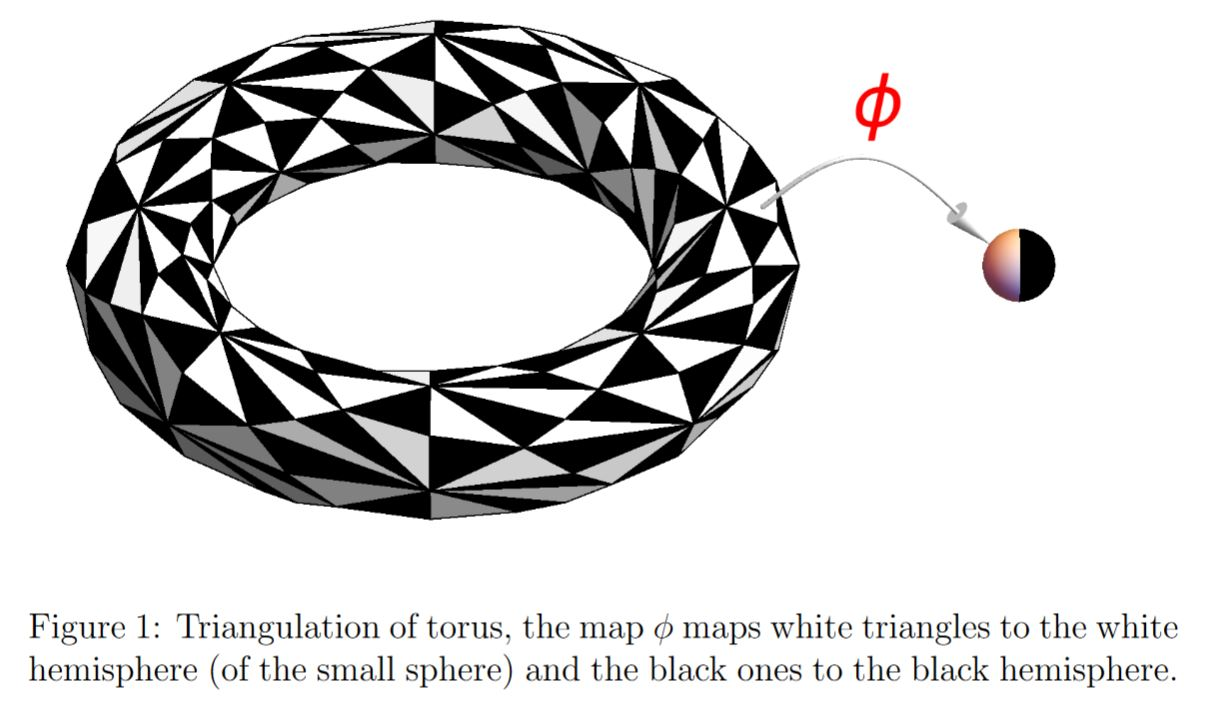Contents

Ingredients

Concepts

Constructions

Examples

Theorems

bundles

# Contents

## Idea

A “branched” or “ramified” covering space is much like a plain covering space, only that over suitable subspaces, such as isolated points, in the base space – called the branch points or ramification points – sheets of the covering may merge, hence that the group of cover automorphisms has fixed points here.

The archetypical examples are in complex analysis and here the most archetypical example of all is the graph of (any choice of) the square root function on the complex plane, which is a double cover away from the point at the origin, but including the origin it is a branched double cover with branch point that origin

Dually branching is reflected in rings of functions by ramification of ideals.

Notably under the function field analogy one may also understand ramification of ideals in number fields as encoding branched coverings (“over Spec(F1)”)

## Examples

### Branched covers of $n$-spheres

Every $n$-dimensional PL manifold admits a branched covering of the n-sphere (Alexander 20).

By the Riemann existence theorem, every connected compact Riemann surface admits the structure of a branched cover by a holomorphic function to the Riemann sphere. See there at branched cover of the Riemann sphere.graphics grabbed from Chamseddine-Connes-Mukhanov 14, Figure 1, Connes 17, Figure 11

For 3-manifolds branched covering the 3-sphere see (Montesinos 74).

All PL 4-manifolds are simple branched covers of the 4-sphere (Piergallini 95, Iori-Piergallini 02).

But the n-torus for $n \geq 3$ is not a cyclic branched over of the n-sphere (Hirsch-Neumann 75)

### Of arithmetic schemes

• Jürgen Neukirch, Algebraische Zahlentheorie (1992), English translation Algebraic Number Theory, Grundlehren der Mathematischen Wissenschaften 322, 1999 (pdf)

### Of manifolds

Every $n$-dimensional PL manifold is a branched cover of the n-sphere:

For 3-manifolds branched covering the 3-sphere see

• J. Montesinos, A representation of closed orientable 3-manifolds as 3-fold branched coverings of $S^3$, Bull. Amer. Math. Soc. 80 (1974), 845-846 (Euclid:1183535815)

All PL 4-manifolds are simple branched covers of the 4-sphere:

The n-torus for $n \geq 3$ is not a cyclic branched over of the n-sphere: